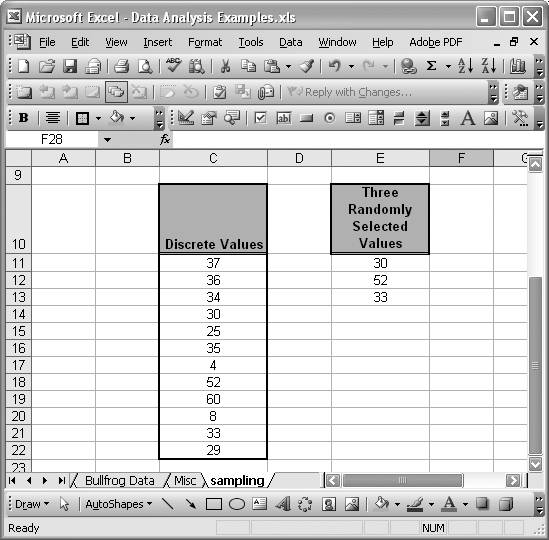# Sampling Data

Problem

You need to draw random samples from a finite population of discrete values.

Solution

Use the Analysis ToolPak Sampling tool. Select Tools [Image_Link]/images/U2192.jpg border=0> Data Analysis from the main menu bar and then select Sampling from the list of available tools.

Discussion

Figure 5-22 shows a spreadsheet containing a set of discrete values from which three were selected at random. The Sampling tool discussed a moment ago was used to draw these three random samples.

Upon selecting the Sampling tool from the Data Analysis tool list, you should see the Sampling dialog box shown in Figure 5-23.

Figure 5-22. Sampling discrete valuesFigure 5-23. Sampling dialog boxIn the Input Range, enter or select the cell range containing the discrete values from which to draw random samples. Next, select the Sampling Method, which can be either Periodic or Random. If you choose Periodic you also have to specify a periodic interval where sampling will take place. At every kth period, a random sample will be drawn. For example, if you choose Periodic with a period of 2 for the example data shown in Figure 5-22, then six samples will be drawn, corresponding to every other value in the input range starting from the second value.

If you choose Random, then you have to specify how many random samples to draw. In this example, I chose to draw three random samples. Finally, select your output options and press OK. In this example I chose to display the results in the same worksheet, starting at cell E11. The results are shown in Figure 5-22.

See Recipe 5.8 to learn how to generate random numbers in Excel.Excel Scientific and Engineering Cookbook (Cookbooks (OReilly))
ISBN: 0596008791
EAN: 2147483647
Year: N/A
Pages: 206
Authors: David M Bourg